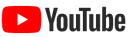Concept Lesson Subtracting Mixed Numbers with RenamingMath 7, Lesson 4-5 Subtracting Mixed Numbers (using rectangles to illustrate renaming)Math 7, Lesson 4-5 Add and Subtract Like FractionsMath 7, Lesson 4-3 Compare and Order Rational NumbersMath 7, Lesson 4-2 Terminating and Repeating DecimalsMath 7, Lesson 4-1

 Concept Lesson Multiplying and Dividing IntegersMath 7A, Lesson 2-4 and 2-5 Math 7, Lesson 3-4 and 3-5 Subtracting Integers by Adding the OppositeMath 7A, Lesson 2-3 Math 7, Lesson 3-3 Adding Integers – “Which do you have more of?” methodMath 7A, Lesson 2-2 Math 7, Lesson 3-2